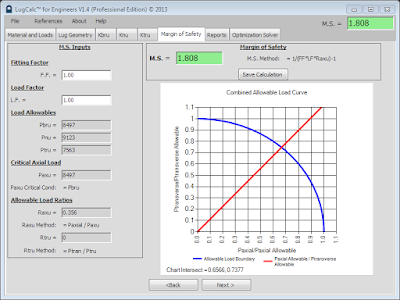# Margin of Safety

The margin of safety is a theoretical unitless value used in failure theory to describe how close a design is to maximizing static strength capacity. It is generally the allowable strength divided by the actual load minus one. Therefore, if a design can withstand 100 lbs and 100 lbs is applied, 100/100-1, the result is 0. When the result is 0, it means the structure is reacting as much load as it can withstand. Generally, the design should always have a margin of safety greater than zero. LugCalc™ calculates the allowable load a lug joint can withstand, divides this by the actual load, and subtracts one. In practice, the exact method utilizes a stress combination equation to account for combined loading and other factors. Please read the LugCalc™ references for a detailed discussion of  how margin of safety is calculated using combination equations. Margin of safety is output to the M.S. = box. The box background is green for positive values and red for negative values.Margin of Safety Screen Shot

Margin of safety is a function of numerous inputs.

Fitting Factor: Fitting factor is a knockdown value applied to a margin of safety calculation to account for variability and unknowns in the design. The unknowns may include material properties, grain direction, manufacturing variability, residual stresses, or any other physical condition which may negatively impact design strength. Governments and OEMs have generally recommend fitting factors in the range of 1.15 – 1.25 as a minimum in design. The fractional component of the fitting factor number represents the percentage of knockdown applied.

Load Factor: Load factor is identical to fitting factor in function but addresses load variability instead of design variability. If it was suspected that a load condition may increase by 25% at some point in the future and the designer or engineer needs to account for this, a load factor of 1.25 would then be applied.

Load Allowables: Load allowables are the calculated maximum load the lug joint can withstand with respect to the three failure modes. These values are the result of the calculations of the preceding tabs in LugCalc™.

Critical Axial Load: Critical axial load is the lowest of the Pbru and Pnu allowables. It is the dominant failure mode of the lug geometry in tension. Therefore, if Pbru is the lowest value, it would be expected the lug fail in bearing tension before it fails in net section tension. To calculate the margin of safety, only the lowest of Pbru or Pnu is used.

Allowable Load Ratios: Allowable load ratios are the fractional result of dividing the allowable tension load by the actual and the allowable transverse by the actual.

Margin of Safety: Margin of safety is then calculated using a combination equation that accounts for all the previously discussed factors. To learn more about the specific method of calculation, please read the LugCalc™ references.

Combined Allowable Load Curve: This curve represents the theoretical maximum combined (axial and transverse) load the lug joint can support. The percentages are derived from the intersect of allowable slope curve with a unit one curve. The values of the intersect are printed at the bottom of the graph. These values will help you quickly determine how over-designed the joint may be. This curve is only applicable if you have combined axial and transverse loads (oblique loading) acting on the lug.

It is strongly recommended the user become familiar with the workings of LugCalc™ when calculating the margin of safety value. This familiarization can be obtained by reading through the LugCalc™ reference material.